# FILTER function in ExcelFiltering is a common everyday action for most Excel users.  Whether using AutoFilter or a Table, it is a convenient way to view a subset of data quickly.  Until the FILTER function came to Excel, there was no easy way to achieve this with formulas.  When Microsoft announced the changes to Excel’s calculation engine, they also introduced a host of new functions.  One of those new functions is FILTER, which returns all the cells from a range that meet specific criteria.

At the time of writing, the FILTER function is only available to those on a Microsoft 365 subscription.  It will not be available in Excel 2019 or earlier versions.

I recommend you download the files which support this post, as you’ll be able to work along with examples.  This is the best way to learn.  You’ll be able to see the solutions in action, plus the file will be useful for future reference.  The support files are available for FREE to newsletter subscribers.

• My favorite tips and tricks direct to your inbox
• Exclusive content (which is only available to subscribers)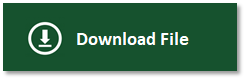The filename for this post is 0035 FILTER function in Excel.zip

Watch the video:

Contents

## Arguments of the FILTER function

Before we look at the arguments required for the FILTER function, let’s look at a basic example to appreciate what it does.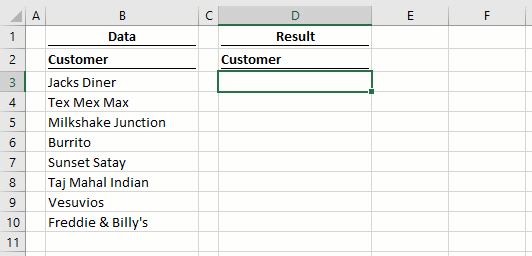Here the FILTER function returns all the values in cells B3-B10 where the number of characters is greater than 15.  Not a scenario that many of us will need, but it demonstrates the power of the new FILTER function.

FILTER has three arguments:

`=FILTER(array, include, [if_empty])`
• array: The range of cells, or array of values to filter.
• include: An array of TRUE/FALSE results, where the TRUE values will be retained in the filter.
• [if_empty]: The value to display if no rows are returned.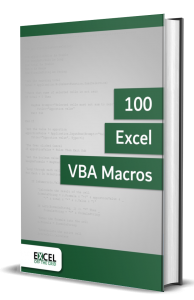• Contains 100 Excel VBA macros
• Learn VBA by following along with the example codes
• Apply to your macros, automate Excel, save time.## Examples of using the FILTER function

The following examples illustrate how to use the FILTER function.

### Example 1 – FILTER returns an array of rows and columns

In this example, cell F3 contains a single formula, but this formula returns an array of values into the neighboring rows and columns.The formula in cell F3 is:

`=FILTER(B3:D10,C3:C10>100)`

This single formula is returning 2 rows and 3 columns of data where the values in C3-C10 are higher than 100.

### Example 2 – #CALC! error caused by the FILTER function

The screenshot below displays what happens when the result of the FILTER function has zero results – we get the new #CALC! error.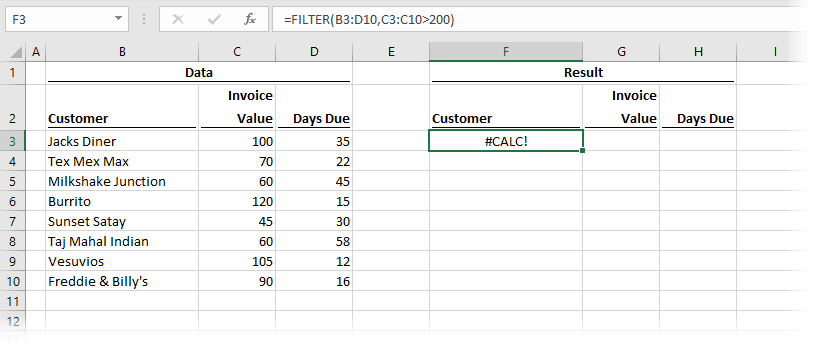The formula in cell F3 is:

`=FILTER(B3:D10,C3:C10>200)`

As no rows meet the criteria of Invoice Value being higher than 200), the FILTER cannot return a value, so the #CALC! error is displayed.

Thankfully, Microsoft has given us the if_empty argument, which displays a message if there are no rows returned.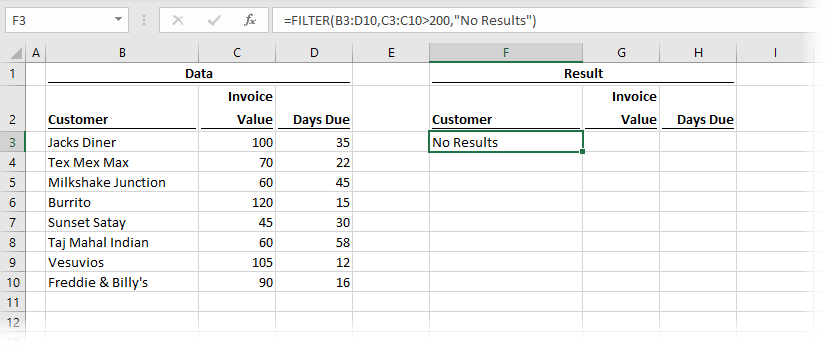The formula in cell F3 is:

`=FILTER(B3:D10,C3:C10>200,"No Results")`

If we wanted to display a result in each column, we could include a constant array within the if_empty argument.  The following would show n/a in the Invoice Value and Days Due columns.

`=FILTER(B3:D10,C3:C10>200,{"No Results","n/a","n/a"})`

This formula would result in the following: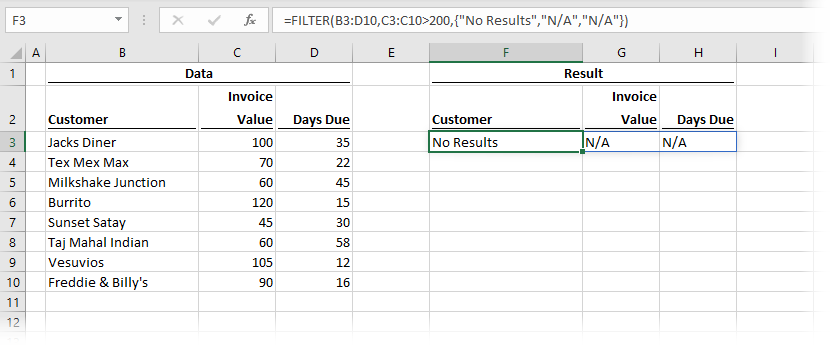### Example 3 – FILTER expands automatically when linked to a table

This example shows how the FILTER function responds when linked to an Excel table.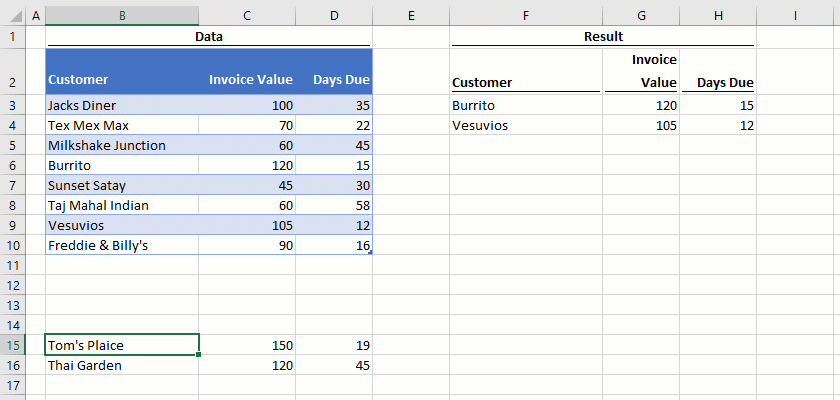New records added to the table which meet the criteria are automatically added to the spill range of the function.  Amazing stuff!

### Example 4 – Using FILTER with multiple criteria.

Example 4 shows how to apply FILTER with multiple criteria.The formula in cell F3 is:

`=FILTER(B3:D10,(C3:C10>50)*(D3:D10>30))`

For anybody who has used the SUMPRODUCT function, this method of applying multiple conditions will be familiar.  Multiplication creates AND logic (i.e., all the criteria must be TRUE).  The example above shows where the Invoice Value is greater than 50 and the Days Due is greater than 30.

Addition creates OR logic (i.e., any individual condition can be TRUE).The formula in cell G3 is:

`=FILTER(B3:D10,(C3:C10>50)+(D3:D10>30))`

The example above shows where the Invoice Value is greater than 50 or the Days Due is greater than 30.

### Example 5 – Using FILTER for dependent dynamic drop-down lists

Drop-down lists are a data validation technique.  Dependent drop-down lists are an advanced technique where the lists change depending on the result of another cell.  For example, if the first drop-down list displays country names, the second drop-down list should only display cities that exist in that country.  There are currently very tedious methods to achieve this effect, but the new FILTER makes this super easy.The formula in cell H3 is:

`=UNIQUE(B3:B10)`

The UNIQUE function creates a unique list to populate the drop-down in cell F4.

The formula in cell I3 is:

`=FILTER(C3:C10,B3:B10=F4)`

Depending on the value in cell F4, the values returned by the FILTER function change.  The second drop-down in cell F6 changes dynamically based on the value in Cell F4.

### Example 6 – Using FILTER with other functions

In this final example, FILTER is nested inside the SORT function.The formula in cell F3 is:

`=SORT(FILTER(B3:D10,D3:D10<=30))`

First, the FILTER function returns the cells based on the Days Due being less than or equal to 30.  The SORT function then puts the Customers into ascending alphabetical order.

There is a lot to learn about dynamic arrays and the new functions.  Check out my other posts here to learn more:

Don’t forget: